• 基础训练BASIC-13 数列排序 n = int(input()) list = list(map(int,input().split())) list.sort() for i in range(0,len(list)): print(str(list[i]),end=" ")
基础训练BASIC-13 数列排序
n = int(input())
list = list(map(int,input().split()))
list.sort()
for i in range(0,len(list)):
print(str(list[i]),end=" ")

展开全文• 蓝桥杯数列排序问题python实现 题目 问题描述 给定一个长度为n的数列，将这个数列按从小到大的顺序排列。1<=n<=200 输入格式 第一行为一个整数n。 　第二行包含n个整数，为待排序的数，每个整数的绝对值小于...
• 问题描述 　给定一个长度为n的数列，将这个数列按从小到大的...　输出一行，按从小到大的顺序输出排序后的数列。 样例输入 5 8 3 6 4 9 样例输出 3 4 6 8 9 这道看似简单的题纠结了我好久，，，一边欣赏自己...
问题描述
给定一个长度为n的数列，将这个数列按从小到大的顺序排列。1<=n<=200
输入格式
第一行为一个整数n。 　　第二行包含n个整数，为待排序的数，每个整数的绝对值小于10000。
输出格式
输出一行，按从小到大的顺序输出排序后的数列。
样例输入
5 8 3 6 4 9
样例输出
3 4 6 8 9
这道看似简单的题纠结了我好久，，，一边欣赏自己简洁的语法，一边郁闷着，到底是哪里写的不对，通过不了系统测试。后来，在一位朋友的指导下，才发现自己没有注意到题目中的两个限制条件，1<=n<=200和每个整数的绝对值小于10000。限制条件很重要！做题要仔细！人生苦短，谨防踩坑！
下面是代码：）
x = int(input())
if x >= 1 & x <= 200:
list1 = list(map(int, input().split()))
list1.sort()
for num in range(0, x):
if abs(list1[num]) < 10000:
print(list1[num], end=" ")

展开全文• Python- 数列排序 资源限制 时间限制：1.0s 内存限制：512.0MB 问题描述 给定一个长度为n的数列，将这个数列按从小到大的顺序排列。1<=n<=200 输入格式 第一行为一个整数n。 第二行包含n个整数，为...
蓝桥杯大赛 基础练习
Python- 数列排序
资源限制

时间限制：1.0s 内存限制：512.0MB

问题描述

给定一个长度为n的数列，将这个数列按从小到大的顺序排列。1<=n<=200

输入格式

第一行为一个整数n。 第二行包含n个整数，为待排序的数，每个整数的绝对值小于10000。

输出格式

输出一行，按从小到大的顺序输出排序后的数列。

样例输入

5 8 3 6 4 9

样例输出

3 4 6 8 9

代码示例
n = input()
x = input()
m = []
m = x.split()
for i in range(0,int(n)):
m[i] = int(m[i])
m = sorted(m)
for i in range(0,int(n)):
print(m[i],end=" ")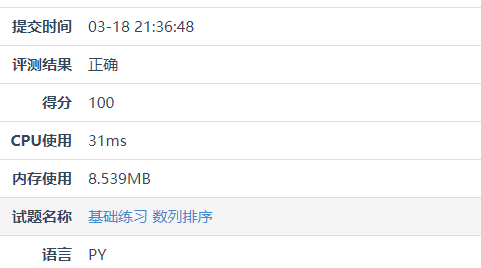展开全文算法 数据结构 经验分享 程序人生
• 基础练习BASIC-13 数列排序BASIC-12 十六进制转八进制BASIC-11 十六进制转十进制BASIC-10 十进制转十六进制BASIC-9 特殊回文数BASIC-8 回文数BASIC-7 特殊的数字BASIC-6 杨辉三角形BASIC-5 查找整数BASIC-4 数列特征...
• 今天使用蓝桥杯系统时发现一个问题,同样的代码,封装和不封装在蓝桥杯评测系统运行时是不一样的, 首先是不封装运行的数据如下: 然后是封装后运行的数据如下: 可以看到,虽然封装后的占用内存稍大于不封装的,但是封装...
小提示:
今天使用蓝桥杯系统时发现一个问题,同样的代码,封装和不封装在蓝桥杯评测系统运行时是不一样的,
首先是不封装运行的数据如下: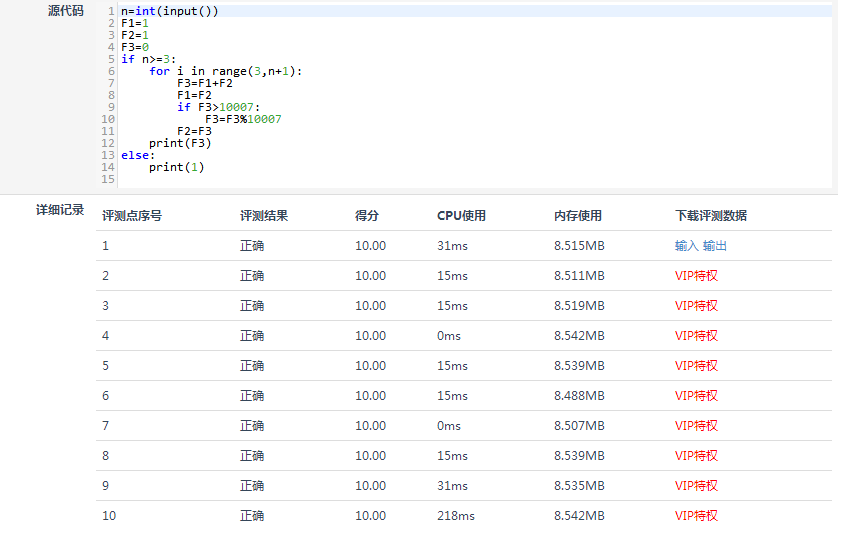然后是封装后运行的数据如下: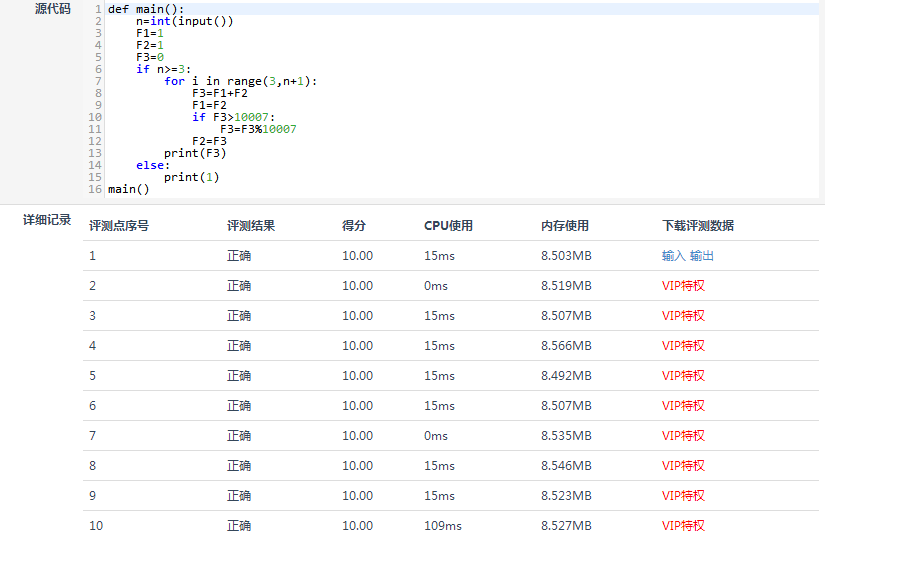可以看到,虽然封装后的占用内存稍大于不封装的,但是封装后的运行速度明显比不封装的快
题目要求:
时间限制：1.0s 内存限制：256.0MB 问题描述 Fibonacci数列的递推公式为：Fn=Fn-1+Fn-2，其中F1=F2=1。
当n比较大时，Fn也非常大，现在我们想知道，Fn除以10007的余数是多少。
输入格式 输入包含一个整数n。 输出格式 输出一行，包含一个整数，表示Fn除以10007的余数。 说明：在本题中，答案是要求Fn除以10007的余数，因此我们只要能算出这个余数即可 ，而不需要先计算出Fn的准确值，再将计算的结果除以10007取余数，直接计算余数往往 比先算出原数再取余简单。
样例输入 10 样例输出 55 样例输入 22 样例输出 7704 数据规模与约定 1 <= n <= 1,000,000。
代码图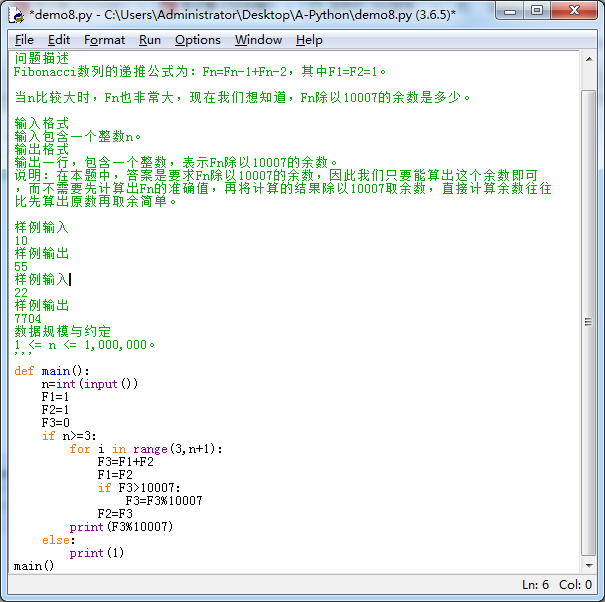代码如下
'''
时间限制：1.0s   内存限制：256.0MB
问题描述
Fibonacci数列的递推公式为：Fn=Fn-1+Fn-2，其中F1=F2=1。

当n比较大时，Fn也非常大，现在我们想知道，Fn除以10007的余数是多少。

输入格式
输入包含一个整数n。
输出格式
输出一行，包含一个整数，表示Fn除以10007的余数。
说明：在本题中，答案是要求Fn除以10007的余数，因此我们只要能算出这个余数即可
，而不需要先计算出Fn的准确值，再将计算的结果除以10007取余数，直接计算余数往往
比先算出原数再取余简单。

样例输入
10
样例输出
55
样例输入
22
样例输出
7704
数据规模与约定
1 <= n <= 1,000,000。
'''
def main():
n=int(input())
F1=1
F2=1
F3=0
if n>=3:
for i in range(3,n+1):
F3=F1+F2
F1=F2
if F3>10007:
F3=F3%10007
F2=F3
print(F3%10007)
else:
print(1)
main()

运行效果图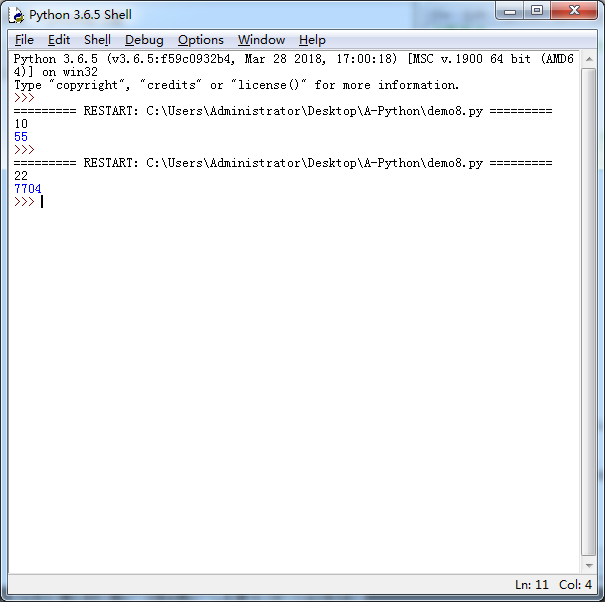展开全文开发语言
• 题目要求: 资源限制 时间限制：1.0s 内存限制：512.0MB 问题描述 　给定一个长度为n的数列，将这个数列按从小到大的顺序...　输出一行，按从小到大的顺序输出排序后的数列。 样例输入 5 8 3 6 4 9 样例输出 3 4 6 8...
• 试题 基础练习 数列排序 目录题目资源限制问题描述输入格式输出格式样例输入样例输出解答Python源代码： 题目 资源限制 时间限制：1.0s 内存限制：512.0MB 问题描述 给定一个长度为n的数列，将这个数列按从小到...算法
• 数列排序 试题 基础练习 资源限制 时间限制：1.0s 内存限制：512.0MB 问题描述 　给定一个长度为n的数列，将这个数列按从小到大的顺序排列。1<=n<=200 输入格式 　第一行为一个整数n。 　第二行包含n个整数...算法
• 第一版写于2020-11-13，第十一届蓝桥杯国赛前夕，老师和同学建议说写博文可以让学习更高效，陆陆续续写一些题解和学习笔记，在我的代码中我加了很多有助于理解程序...数列排序 十六进制转八进制 十六进制转十进制 ...数据结构与算法 算法
• 描述： 给定一个长度为n的数列，将这个数列按...　输出一行，按从小到大的顺序输出排序后的数列。 输出样例: 3 4 6 8 9 代码如下: #代码1： n = int(input()) arr = list(map(int, input().split())) arr.sort() f算法
• 蓝桥杯 Python 基础练习题 问题描述 　给定一个长度为n的数列，将这个数列按从小到大的顺序排列。1<=n<=200 输入格式 　第一行为一个整数n。 　第二行包含n个整数，为待排序的数，每个整数的绝对值小于10000。...
• 问题描述 　给定一个长度为n的数列，将这个数列按从小到大...　输出一行，按从小到大的顺序输出排序后的数列。 样例输入 5 8 3 6 4 9 样例输出 3 4 6 8 9 知识点：A.sort()函数 可以将列表从小到大排序 思路：直接将...算法
• 十二届蓝桥杯Python组省赛试题及解析 A题：卡片 B题：直线 C题：货物摆放 D题：路径 E题：回路计数 F题：时间显示 G题：杨辉三角形 H题：左孩子右孩子 I题：异或数列 J题：括号序号 先把省赛试题分享出来，再慢慢...
• 蓝桥杯 定义二维列表： 方法一 a = [[i for i in range(10)] for j in range(10)] # 10行10列，每一行内容为[0, 1, 2, 3, 4, 5, 6, 7, 8, 9] print(a) print('=' * 80) b = [[0 for i in range(10)] for j in range...
• 蓝桥杯基础练习-数列排序Python） 这道题考排序，总体难度不大，不多说直接上代码： n=int(input()) dp=[int(i) for i in input().split()] dp.sort() print(' '.join(str(i) for i in dp))
• 数列排序 资源限制 时间限制：1.0 s 内存限制：512.0 MB 问题描述 给定一个长度为n的数列，将这个数列按从小到大的顺序排列。1<=n<=200 输入格式 第一行为一个整数n。 　第二行包含n个整数，为待排序的数，每...c++ 算法
• n = input() num1 = input().split() num2 = list(map(int, num1)) if len(num2) == int(n): num2.sort() for i in num2: print(i, end=' ')算法
• 题目 问题描述 给出n个数，找出这n个数的最大值，最小值，和。 输入格式 第一行为整数n，表示数的个数。 第二行有n个数，为给定的n个数，每个数的绝对值...Python代码 while True: try: a=int(input()) b=input算法
• 1、先将输入的等差数列的数字存入数组中，并对其进行排序 2、计算邻近数值的差，并记录最小的差值，为等差数列的差 3、用最大的项除以最小的项，然后加1,就是一共有多少项 n=int(input()) ls=list(map(int,input()....算法
• Python 蓝桥杯试题 基础练习 数列排序 题目描述： 问题描述 给定一个长度为n的数列，将这个数列按从小到大的顺序排列。1<=n<=200 输入格式 第一行为一个整数n。 第二行包含n个整数，为待排序的数，每个整数...
• 蓝桥杯 试题 基础练习 数列排序 资源限制 时间限制：1.0s 内存限制：512.0MB 问题描述 　给定一个长度为n的数列，将这个数列按从小到大的顺序排列。1<=n<=200 输入格式 　第一行为一个整数n。 　第二行包含n个...算法
• 我的代码： n=int(input()) a=input() if 1<=n and n<=200: b=list(map(int,a.split())) b.sort() for i in b: if abs(i)<10000: print(i,end=' ') 参考代码： x = int(input()) ... ..
• 2019 第十届蓝桥杯C/C++ 省赛B组题解 1. 试题 A: 组队 可以考虑使用 Excel 进行辅助计算。 答案：490 2. 试题 B: 年号字串 特殊的进制转换——十进制转二十六进制 27%26=1……1（即 AA）28%26=1……2（即 AB）329%...算法...

# 蓝桥杯python数列排序python 订阅